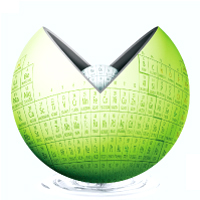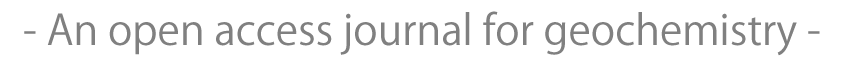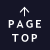JavaScript requeired.Published for geochemistry community from Geochemical Society of Japan.
Note

## Radon exhalation from the Upper Silesian coal ashes

A. T. SOLECKI, D. E. TCHORZ-TRZECIAKIEWICZ
Geochemical Journal, Vol. 45, No. 6, P. 491-496, 2011

#### ABSTRACT

The radon exhalation has been measured for samples of coal ashes by the can technique. In this technique, the ratio of the radon content in the air inside the can and the total radium content of the sample is often regarded to be the approximation of the emanation coefficient. In fact it is the exhalation coefficient, measure of the exhalation from the sample, and depends strongly on the sample size and geometry. Analysis of the relation between the defined exhalation coefficient and the sample thickness resulted in the exponential function. The exponential function for thickness approaching zero results in exhalation coefficient equal 13%, which can be treated as an approximation of emanation coefficient.

#### KEYWORDS

radon exhalation, coal ashes, emanation coefficient, exhalation coefficient, Poland

## All Issues

Current Issue:
Stats:
Impact Factor: 0.8 (2022)
Submission to final decision: 9.6 weeks (2022)